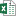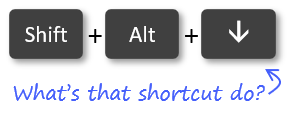# The SUBTOTAL Function for Excel Tables Total Row Explained

Bottom Line: Learn how the SUBTOTAL function is used in the formulas in the Total Row of Excel Tables.

Skill Level: Beginner

## Video Tutorial

You can follow along with the video using the same workbook I do, if you like. Here is the Excel file:SUBTOTAL Function Explained (26.4 KB)

## Using the SUBTOTAL Function in Excel

The SUBTOTAL function is a versatile function that can be used in many different scenarios in Excel.

For this post, I explain how the SUBTOTAL function is used in the Total Row of Excel Tables. The total row automatically creates the formula for us, but it's important to understand how the SUBTOTAL function works.

Turning the Total Row on and off for an Excel Table is easy. Just click anywhere inside the table, go to the Design tab, and check or uncheck the box for Total Row.

In the next post I explain a different use for SUBTOTAL in more of an outline or report style format. Sometimes you will see subtotal rows interspersed throughout a report or a data set, and then a Grand Total row at the very bottom. I will be discussing how the SUBTOTAL function and the Subtotal Feature of Excel help with that in the next post.

## The SUBTOTAL Function Explained

This SUBTOTAL function will only display the totals for any visible cells. If you filter out or manually hide rows, the values from those cells will automatically be removed from the sum that the SUBTOTAL function creates.

The SUBTOTAL Function has two arguments.

1. The first argument identifies what type of calculation you are looking for, such as an average, a sum, a count, a standard deviation, etc. Each of these types of calculation is assigned a number by Excel. 1 through 11 calculate based on all of the cells in a given data range, while 101 through 111 calculate only the cells that are visible in a data range. The default for the Total Row is 109, which is the designation for a sum of the visible cells.
1. The second argument is the reference. This is simply the data range that you want the subtotal to calculate from. You can include more than one reference if you want the subtotal to include data from non-contiguous ranges.

## Quickly Changing the Calculation Type

Next to the Subtotal calculation is a little arrow that expands to a drop-down box. This menu allows you to select a different type of calculation.

Instead of clicking the down arrow, you can use the keyboard shortcut Alt + .

## AutoSum

Another way to create a SUBTOTAL function is by clicking on the AutoSum button on the Home tab.

Or you can use the keyboard shortcut Alt + =.

This calculates the same as the default for a Total Row (109), which is a sum of all visible cells in the referenced range. The only quirk about using AutoSum is that it doesn't format the result using the same formatting as the reference range.

## Related Posts

Check out these related videos and tutorials for more information about the SUBTOTAL function or Excel Tables.

The Excel SUBTOTAL Function – Calculations on Filtered & Visible Cells Only

The SUBTOTAL Metrics Macro – Create a Summary Table of All Function Types

Create SUBTOTAL Formulas with the AutoSum Button or Keyboard Shortcut

Excel Tables Tutorial Video – Beginners Guide for Windows & Mac

Best Practices for Naming Excel Tables

## Conclusion

I hope this post has been valuable in helping you understand how the Total Row works in Excel Tables. I welcome any feedback or questions in the comments below. See you in the next post!

•Ken says:

Why / When would use the ‘Aggregate’ function instead of the ‘SubTotal’?

•Jon Acampora says:

Hey Ken,

Great question! The AGGREGATE function was introduced in Excel 2010. It is similar to SUBTOTAL, but has a few additional options and calculation types.

AGGREGATE has an option to ignore cells that contain errors, which is something SUBTOTAL can’t do. It can also ignore other SUBTOTAL and AGGREGATE functions.

AGGREGATE also has 8 additional calculation types including: MEDIAN, MODE.SNGL, LARGE, SMALL, PERCENTILE.INC, QUARTILE.INC, PERCENTILE.EXC, and QUARTILE.EXC.

I’ll do a post in the future on AGGREGATE. It’s a great function to know. SUBTOTAL is probably used more frequently because it is built into Tables and used by the Subtotal feature, which I’ll explain in next week’s post.

I hope that helps. Thanks again and have a nice day! 🙂

•John Garrett says:

Is there a way to select the lowest 10 scores out of the last 20 entries in an array > 20 entries (to calculate a golf handicap)?

•Mark says:

Didnt know of this but will be of use to me, thank you.

•Jon Acampora says:

Awesome! Thanks Mark!

•Viney says:

Thank you for another helpful video. Brilliant!

• Generic filters
Exact matches onlyLearn 10 great Excel techniques that will wow your boss and make your co-workers say, "how did you do that??"

#### Excel Shortcuts ListLearn over 270 Excel keyboard & mouse shortcuts for Windows & Mac.

Excel Shortcuts List